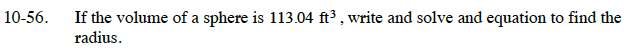Home > ACC7 > Chapter cc310 > Lesson cc310.1.4 > Problem10-56

10-56.

If the volume of a sphere is 113.04 ft3, write and solve and equation to find the radius. Homework Help ✎$\text{The\: formula\: to\: find\: the\: volume\: of\: a\: sphere\: is \:} V = \frac{4}{3}\pi\:r^3.$

How would you undo an exponent?

$113.04\; =\; \frac{4}{3}\pi {\it r}^3$

$\frac{\left(113.04\right)\left(3\right)}{4\pi }={\it r}^3$

$26.99\; \approx\; {\it r}^3$

$\sqrt{26.99}\approx\; \it r$

$3 \approx \it r$

The radius is 3 ft.